Home / Expert Answers / Computer Science / which-of-the-following-is-correctly-describes-methods-of-sorting-data-by-columns-quantitative-vari-pa793

# (Solved): Which of the following is correctly describes methods of sorting data by columns: quantitative vari ...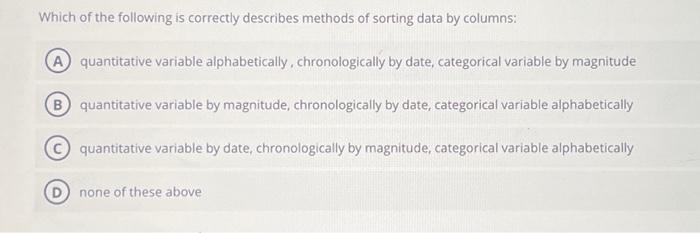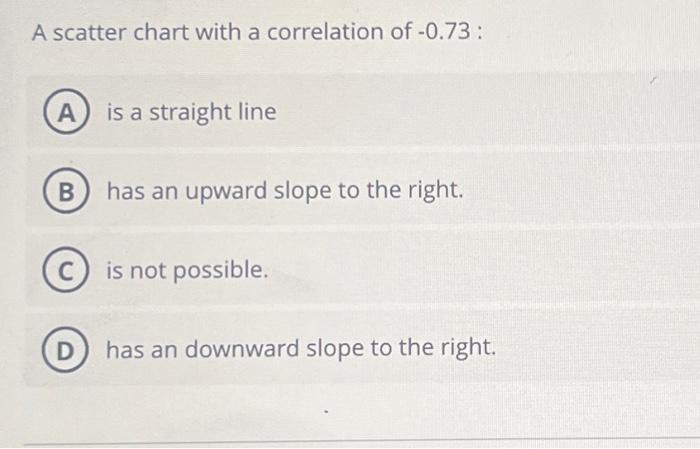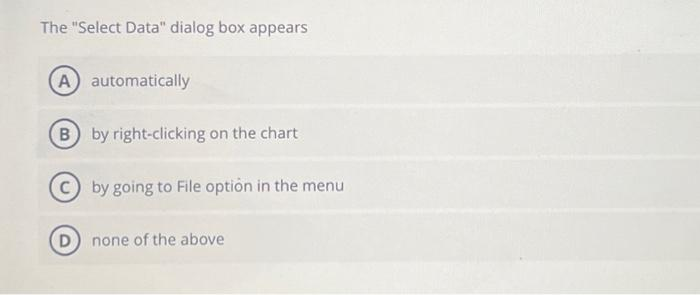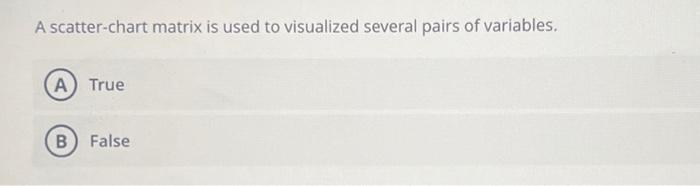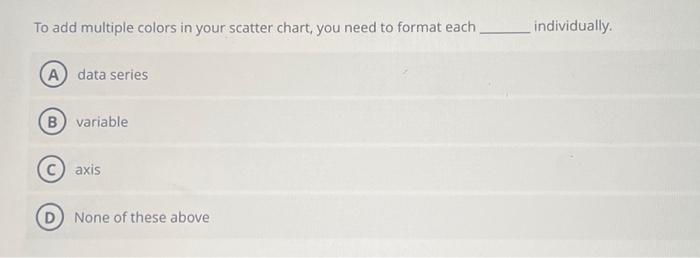Which of the following is correctly describes methods of sorting data by columns: quantitative variable alphabetically, chronologically by date, categorical variable by magnitude quantitative variable by magnitude, chronologically by date, categorical variable alphabetically quantitative variable by date, chronologically by magnitude, categorical variable alphabetically none of these above A scatter chart with a correlation of -0.73 : is a straight line has an upward slope to the right. is not possible. has an downward slope to the right. The "Select Data" dialog box appears automatically by right-clicking on the chart by going to File option in the menu none of the above A scatter-chart matrix is used to visualized several pairs of variables. True False

We have an Answer from Expert